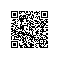# Lambda Calculus

Lambda Calculus是非经典逻辑中的一种，形式比图灵机模型和一阶谓词逻辑等简洁优雅许多，是函数式编程语言的理论支柱，本文主要简单梳理了untyped Lambda Calculus以及Church数的构造。

Functional Programming Languages

• Properties
• based-on lambda calculus
• closure(functor) and high-order function
• lazy evaluation
• recursion
• reference transparently
• no side-effects
• expression

Lambda Calculus

• Four core components

• expression
• variable(value)
• function
• application
• Grammar

• (expression) := (variable) | (function) | (application)
• (function) := lambda (variable).(expression)
• (application) := (expression)(expression)
• examples
• function definition : lambda x.x ==> Identity function I
• function application : (lambda x.x)(y) = y
• free and bound variables

• lambda x.xy ==> x bound but y free
• substitution and reduction

• alpha substitution
• beta reduction
• numbers definition(Church numbers)

• S : lambda wyx.y(wyx) (Successor function)
• 0 : lambda sz.z
• 1 : lambda sz.s(z)
• S(0) = (lambda wyx.y(wyx))(lambda sz.z)
= lambda yx.y((lambda sz.z)(y)x)
= lambda yx.y(x) = 1
• 2 : lambda sz.s(s(z))
• S(1) = (lambda wyx.y(wyx))(lambda sz.s(z))
= lambda yx.y((lambda sz.s(z))yx)
= lambda yx.y((lambda z.y(z))x)
= lambda yx.y(y(x)) = 2
• 3 : lambda sz.s(s(s(z)))
• 3(Func)(var) ==> apply 3 Func times on var

• ’+’ : lambda wyx.y(wyx) (successor function)
• 1 + 2 = 1S(2)
• (lambda sz.s(z)) (lambda wyx.y(wyx)) (lambda ab.a(a(b))) = (lambda z.(lambda wyx.y(wyx))(z)) (2)
= (lambda zyx.y(zyx))(2) = S(2)
• 2 + 2 = 2S(2)
• (lambda sz.s(s(z))) (lambda wyx.y(wyx)) (2) = (lambda z.S(S(z)))(2) = S(S(2))
• multiplication

• ’*’ : lambda xyz.x(yz)
• 1*2 = (lambda abc.a(bc))(1,2) = (lambda bc.1(bc))(2)
= (lambda c.1(2(c)))
= (lambda c.(lambda sz.s(z))(lambda sz.s(s(z)))(c))
= lambda c.(lambda cz.c(c(z))) = 2
• Condition

• T : lambda xy.x
• F : lambda xy.y
• logic operation

• && : lambda xy.xyF

• &&(T,T) = (lambda x1y1.x1)(lambda x2y2.x2)(lambda xy.y) = lambda x2y2.x2 = T
• &&(F,Any) = (lambda x1y1.y1)(Any)(lambda xy.y) = lambda xy.y = F
• | : lambda xy.xTy

• |(F,F) = (lambda x1y1.y1)(lambda xy.x)(lambda x2y2.y2) = lambda x2y2.y2 = F
• |(T,Any) = (lambda x1y1.x1)(lambda xy.x)(Any) = lambda xy.x = T
• ~ : lambda x.xFT

• ~(F) = (lambda xy.y)(lambda x1y1.y1)(lambda x2y2.x2) = lambda x2y2.x2 = T
• ~(T) = (lambda xy.x)(lambda x1y1.y1)(lambda x2y2.x2) = lambda x1y1.y1 = F
• conditional test

• Z : lambda x.xF~F ==> T if x==0 else F
• Z(0) = 0F(~F) = (lambda sz.z)F~F = ~F = T
• Z(1) = (lambda sz.s(z))F~F = F(~)F = (lambda xy.y)(~)(F) = IF = F
• predecessor

• p : lambda zxy.xy ==> a pair (x,y)
• Inc : lambda pz.z(S(pT))(pT) ==> increase each element of one pair (x,x-1) -> (x+1,x)
• P : (lambda n.n(In(lambda z.z00)))F
• nP(0) = 0
• equality and inequality

• >= : lambda xy.Z(xPy) [if x>=y return True else False]
• <= : lambda xy.Z(yPx) [if x<=y return True else False]
• = : lambda xy.^(Z(xPy))(Z(yPx))
• recursion

• Y combinator : Y = lambda f.(lambda x.f(xx))(lambda x.f(xx))
= f((lambda x.f(xx))(lambda x.f(xx)))
• Yf = f(Yf) [Yf ==> recursion of f]
• example 1+2+3…+n : f = lambda rn.(Zn)(0)(nS(r(Pn)))
• Yf = f(Yf) = lambda Yfn.(Zn)(0)(nS(Yf(Pn))) ==> Yf recursion

ref:《A Tutorial Introduction to the Lambda Calculus》使用钉钉扫一扫加入圈子
+ 订阅